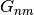Warning

This documents an unmaintained version of NetworkX. Please upgrade to a maintained version and see the current NetworkX documentation.

# gnmk_random_graph¶

gnmk_random_graph(n, m, k, seed=None, directed=False)[source]

Return a random bipartite graph G_{n,m,k}.

Produces a bipartite graph chosen randomly out of the set of all graphs with n top nodes, m bottom nodes, and k edges.

Parameters: n (int) – The number of nodes in the first bipartite set. m (int) – The number of nodes in the second bipartite set. k (int) – The number of edges seed (int, optional) – Seed for random number generator (default=None). directed (bool, optional (default=False)) – If True return a directed graph

Examples

from networkx.algorithms import bipartite G = bipartite.gnmk_random_graph(10,20,50)

gnm_random_graph()
This graph is a bipartite version of therandom graph model.